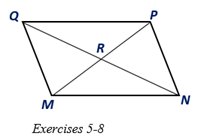Chapter 4.1, Problem 6E### Elementary Geometry for College St...

6th Edition
Daniel C. Alexander + 1 other
ISBN: 9781285195698

#### Solutions

Chapter
Section### Elementary Geometry for College St...

6th Edition
Daniel C. Alexander + 1 other
ISBN: 9781285195698
Textbook Problem
1 views

# For Exercises 5 to 8, MNPQ is a parallelogram with diagonals Q N - and M P - .a) If Q R = 7.3 , find RN. b) If M P = 10.6 , find RP.

To determine

a.

To find:

The length of RN in the parallelogram MNPQ.

Explanation

Given:

MNPQ is a parallelogram with diagonals QN- and MP- and QR=7.3.

Corollary:

The diagonals of a parallelogram bisect each other.

Calculation:

In the given parallelogram MNPQ, given that the diagonals QN- and MP- and QN=12.8

To determine

b.

To find:

The length of RP in the parallelogram MNPQ.

### Still sussing out bartleby?

Check out a sample textbook solution.

See a sample solution

#### The Solution to Your Study Problems

Bartleby provides explanations to thousands of textbook problems written by our experts, many with advanced degrees!

Get Started

#### Find the inverse function of f(x)x2+2forx0.

Calculus: An Applied Approach (MindTap Course List)

#### Multiply: 56748_

Elementary Technical Mathematics

#### Solve the equations in Exercises 126. (x+1)3+(x+1)5=0

Finite Mathematics and Applied Calculus (MindTap Course List)

#### Differentiate the functions in Problems 3-20. 15.

Mathematical Applications for the Management, Life, and Social Sciences

#### (3, 6)

Applied Calculus for the Managerial, Life, and Social Sciences: A Brief Approach

#### True or False: converges conditionally.

Study Guide for Stewart's Multivariable Calculus, 8th

#### What is the goal of a single-case experimental research design?

Research Methods for the Behavioral Sciences (MindTap Course List)